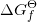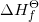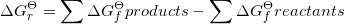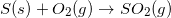## Effective Use of the IB® Chemistry Data Booklet, Part 1

### Effective Use of the IB® Chemistry Data Booklet, Part 1Written by Chris; Chris is available for private tutoring.

IMPORTANT NOTE: to make best use of this blog post, please have a hard copy of the fourth edition of the IB® Data Booklet available to you.

These next 2 blogs will help you with the data booklet during IB® Papers 2 and 3. I’m going to talk about some of the information you may have met in class or read in textbooks and how your data booklet might convey that same information differently; the use of different conventions and nomenclature can lead to problems. I will also write about specific types of questions that require the use of the booklet but not always in the most obvious way.

We’ll work our way through in page order so you can follow with your own version. Make sure it is the most recent (Fourth Edition – First Assessment 2016). Ask your teacher to email you one if you don’t have an electronic version. I won’t be covering every single table in the booklet, only those I feel need addressing.

Contents Page: this might seems obvious, but make sure you are able to navigate the contents page. In particular, take note that some tables contain data on multiple properties. For example, Table 8 contains information on 1st ionization energy, electron affinity and electronegativity, so if you are only scanning page titles you may miss something pertinent. On a similar note, Table 12 is simply described as “Thermodynamic Data”, which is rather vague; however, this table contains some incredibly useful information (see later) and it one of the most used table in exams.

Table 1 – Some Relevant Equations: the main issue here will be whether you have seen or been taught these equations in exactly the same manner using the same format and symbols. Also, be aware that you will not need to be able to use all of these equations; the relevant specification points are given in the left column (which is not true for data booklet in some other Group 4 subjects); HL only begins at 12.1 and you will only be studying one of options A-D.

These are the only equations you are likely to be given so any other you have met along the way will need to be remembered, such as:I have picked out some of the equations in this table that might present some confusion due to possible inconsistencies with the way you learned them in class:

•16.2, 2nd form of Arrhenius equation – the form of the equation given here is typical but has been changed in recent years so it does not now show an obvious similarity to y = mx + c. The equation given can be re-written as:In this form, it is clearly that a plot of ln k vs (1/T) will give a straight line with a gradient of (-Ea/R).

•16.2, 3rd form of Arrhenius equation – this equation is designed for use when data is limited or when the data fall very close to the straight line of best fit. One danger with using this equation over a graphical method is that should you accidentally select two points that are not that close to the line of best fit (and worse still, are on different sides of it) then you will end up with a significant under or overestimation of the activation energy.

•B.7 & D.4, Henderson-Hasselbalch equation – note the form of the equation here appears to be related to acidic buffers (use of pKa and HA). However, it is still possible to use this form of the equation to determine the pH of a basic buffer.

e.g., calculate the pH of a buffer solution made by mixing 200 cm3 of 0.20 mol dm-3 ammonium chloride solution with 250 cm3 of 0.25 mol dm-3 ammonia solution. The two main species present in the buffer are NH3 and NH4+; however you will only find the pKb value for NH3 in the data booklet and no mention of NH4+. The form of the Henderson-Hasselbalch equation given only uses pKa and you cannot simply insert pKb in its place without some other modifications. Therefore, you will need to calculate the pKa of the conjugate acid (NH4+) of the base (NH3) by using pKa = 14 – pKb. In this example, the pKa of NH4+ is 9.25 (14 – 4.75) and this value can go directly into the equation. I will leave you to try to complete the question using this advice.

Make sure you know which species given in a question is A and which is HA. The species A does not have to have a negative charge but simply has one H+ fewer than HA. In the example above, NH3 is A and HA is NH4+.

In a recent paper, students were required to work out the pH of blood based on a hydrogen carbonate-based buffer. However, many were confused as the species given did not appear to be a conjugate acid-base pair; instead the concentrations of aqueous HCO3 and CO2 were given. Students needed to spot that aqueous CO2 is effectively H2CO3 and so acts as HA in the equation. Many students mistook HCO3 for HA as it contains a hydrogen atom.

•C3 & D.8, Nuclear half-life equations – I was surprised to see students mistaking the symbol for half-life (t1/2) for t × ½; you may have used the symbol k in your lessons instead of t1/2.  Also, do not confuse the decay constant (λ) for half-life.

Table 2 – Physical Constants and Unit Conversions: there are a small number of points to clarify here.

•Make sure you know the difference between STP, SATP, standard conditions and standard states. You should have met STP (standard temperature and pressure) in class but you may not have heard of SATP. The conditions for SATP are identical to the commonly used RTP (room temperature and pressure). Standard conditions, which are a ubiquitous part of equations for standard enthalpy changes, are slightly different and no mention is found in Table 2. My advice is that where a definition includes the phrase “under standard conditions” you replace this with “at 298 K and 100 kPa”, which is highly specific.

•Hopefully, you will be aware that equilibrium constants have no units (you do not need to know why). However, the value for Kw in Table 2 gives the units as mol2 dm-6 which is at odds with the IB specification. Ignore the units and do not refer to them or use them in calculations involving Kw, though you should remember the concentrations you calculate using Kw will have units of mol dm-3.

•Table 2 refers to amu in the final entry. You may have used amu in class or you may have used u to signify the unified atomic mass unit. Technically, the term amu is obsolete and was not based on carbon-12. However, many sources still use amu as a term synonymous with u and have re-defined amu For your purposes, amu and u are identical units.

Table 5 – Alphabetical List of the Elements: in the past I have wondered about the purpose of this table but then I forget that students are far less familiar with the periodic table and it is not fair to assume they will know where to look for an element or that they will, intuitively, know the correct symbol. My feeling is that this table is designed to prevent students wasting time searching the periodic table for unfamiliar elements and then ending up confusing, say, yttrium (Y) for ytterbium (Yb). You really shouldn’t be using the wrong symbols for elements given the presence of Table 5.

If a question refers to an element you haven’t heard of or aren’t sure about then do not use the periodic table but use Table 5 to find it (easy since it’s alphabetical). This is particularly helpful when compiling nuclear equations which involve heavy, less common elements such as lutetium, bismuth, etc. Once you’ve identified the correct symbol and atomic number using Table 5 you can then use the periodic table to determine the symbol and atomic number of the products of radioactive decay (because you know where to start, i.e., the atomic number of the starting element and the Periodic Table is arranged in order of atomic number).

Table 6 – The Periodic Table: only a couple of potential issues to address here. First, make sure you pay attention to the two decimal place values given for relative atomic masses. You will be expected to use these values in calculations. Whilst using rounded integer values might not cause too many problems, it certainly has the ability to. Calculations of empirical formulas can be thrown out if 1.00 rather than 1.01 is used for hydrogen and questions that involve determining the identity of an unknown element can yield the wrong element. There are plenty of elements where it is inappropriate to round to a whole number, e.g., Cl, Cu, Mg, Ba, Zn to name but a few. Main advice, use the values provided.

The second issue surrounds the way the data in the periodic table is presented. A key is provided to make it clear that the uppermost number in each entry is the atomic number and the bottommost value is the relative atomic mass (RAM). However, students do get confused especially as the convention for writing this information for an element in, for example, a nuclear equation is:where A is the RAM and Z is the atomic number

Remember, atomic numbers are always integers but RAMs will be averages and hence have decimal places. Also, with the exception of hydrogen, the RAM is always larger than the atomic number.

Table 7 – Melting and Boiling Points: for some reason, these are quoted at 101.325 kPa (one atmosphere) rather than the more common 100 kPa. I think it is safe to assume that the melting and boiling points do not change much between these pressures and so you can easily determine the appropriate states of matter for elements when writing equations for standard enthalpy change of formation.

Table 8 – 1st Ionization Energy, Electron Affinity and Electronegativity: I have mentioned previously that this table contains a mass of data and looks very cramped; what I want to focus on, though, are the electronegativity values and how the IB expects you to use them.

The electronegativity values in Table 8 can be used to determine bond and, therefore, molecular polarity. For a molecule to be polar, the sum of the dipole moments of the individual bonds must be non-zero, i.e., the dipoles must not cancel. This necessarily means the bonds within a polar molecule must also be polar. This is where the difficulty arises because agreement about what makes a bond polar is not universal. Technically, if the atoms in a bond are different elements then the bond will be polar to some extent; however, in many cases the difference is so small as to make little difference to the characteristics of the molecule, i.e., the molecule will still behave as though it is non-polar. Many “definitions” of a polar bond will refer to a “difference of 0.5 to 2.0 units on the Pauling scale” but this is not a fixed rule.

For IB questions, you simply use the one decimal place values given in Table 8. If the values are identical, e.g., P (2.2) and H (2.2) then the bond is non-polar meaning a molecule such as PH3 is also non-polar. The difficulty arises with hydrocarbons that show asymmetry, such as 2-methylpropane. Carbon is more electronegative (2.5) than hydrogen (2.2) and in 2-methylpropane the dipoles do not cancel giving the molecule a net dipole moment. However, the molecular dipole moment is very small as a result of weakly polar (essentially non-polar) C—H bonds. It is safe at this level to assume all unsubstituted hydrocarbons will be non-polar.

Table 10, 11 and 18 – Bond Lengths, Bond and Lattice Enthalpies: my main point here is to be aware that these data are in a format you may not be too familiar with. Typically, you will have been given these data in a tabulated list but the IB Data Booklet uses a matrix table in which each cell corresponds to a combination of two variables listed as row or column headings. Make a note that multiple bonds are not included in the table but beneath it so the table only refers to single bonds.

Table 12 – Thermodynamic Data: there is a lot of information in this table so make sure you know what is here. Often, questions will refer to the data booklet but may not specify the particular table and this one is one of the more commonly used. You should be familiar with using standard enthalpy change of formation and standard entropies but you may not have used standard Gibbs free energy change of formation that much.

Suffice to say that you can create energy cycles forin the same manner as you can for. You can also use the following equation to determine the Gibbs free energy change of a reaction:Also, be aware that water has a multiple entry as both liquid water and steam. The thermodynamic data is different depending on the state of matter. This is especially important when analysing questions that involve non-standard conditions, e.g., combustion of a fuel producing steam.

Table 13 – Enthalpies of Combustion: All I want to point out here is that the standard enthalpy change of combustion of sulfur is also the same as the standard enthalpy change of formation of sulfur dioxide:Table 16 – Ligands: the structure of EDTA shown in Table 16 only shows it as a bidentate ligand (using the lone pair on each of the two nitrogen atoms). However, more typically, EDTA is described as a hexadentate ligand that is able to chelate metal ions. In order to do this, EDTA needs to exist as a tetraanion, i.e., as EDTA4-. To do this, a hydrogen ion dissociates from each of the carboxyl moieties leaving behind four carboxylate groups that can coordinate to a metal ion via the negative charge.

Table 17 – Colour Wheel: just in case you are not sure what you might do with this diagram, the colour wheel is used to determine the likely colour of a solution given information about the photon(s) of light that the solution absorbs. It is simple to use: if you know the colour or wavelength of the photon absorbed then the solution will take on the appearance of the complementary colour to that absorbed which is simply the colour directly opposite in the colour wheel, e.g., a solution absorbs a photon of 660 nm, this falls in the red region and the solution appears green.

You can also work backwards from the colour of the solution, e.g., copper(II)sulfate produces a blue solution, this is complementary to orange so the solution absorbs photons of orange light.

In Part 2 of our blog, which we expect to publish at the end of April (well before your exam!) we will look at the second half of the Data Booklet.

Be sure to give us a shout if you have any questions!

Warp Drive Tutors, Inc. has been developed independently from and is not endorsed by the International Baccalaureate Organization. International Baccalaureate®, Baccalaureat International®, Bachillerato Internacional® and IB® are registered trademarks owned by the International Baccalaureate Organization.